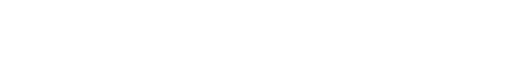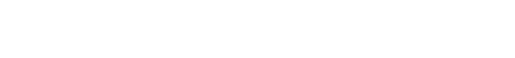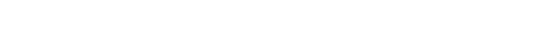# 1.2.2 Mechanical Properties of GaN

1.2.2 Mechanical Properties of GaN

GaNhas a molecular weight of 83.7267 g mol1 in the hexagonalwurtzite structure.The
lattice constant of early samples of GaN showed a dependence on growth conditions,
impurity concentration, and film stoichiometry . These observations were

attributed to a high concentration of interstitial and bulk extended defects. A case in
point is that the lattice constants ofGaNgrown with higher growth rates were found to
be larger. When doped heavily with Zn  and Mg , a lattice expansion occurs
because athighconcentrations thegroupIIelementbegins tooccupythe lattice sites of
the much smaller nitrogen atom. At room temperature, the lattice parameters ofGaN
platelets  prepared under high pressure at high temperatures with an electron
concentration of 5 · 1019 cm3 are a¼3.18900.0003Å and c¼5.18640.0001 Å.
The freestanding GaN with electron concentration of about 1016 cm3, originally
grown on sapphire (0 0 0 1) by hydride vapor phase epitaxy (HVPE) followed by liftoff,
has lattice constants of a¼3.20560.0002Å and c¼5.19490.0002Å. For GaN
powder, a and c values are in the range of 3.1893–3.190 and 5.1851–5.190 Å,
respectively. Experimentally observed c/a ratio for GaN is 1.627, which compares
well with 1.633 for the ideal case, and the u parameter calculated using Equation 1.1 is
0.367, which is very close to the ideal value of 0.375.
For more established semiconductors with the extended defect concentration from
low to very low, such as Si, GaAs, and so on, the effect of doping and free electrons on
the lattice parameter has been investigated rather thoroughly. In bulk GaN grown by
the high-pressure technique, the lattice expansion by donors with their associated free
electrons has been investigated . However, large concentration of defects and
strain, which could be inhomogeneous, rendered the studies of this kind less reliable
in GaN layers. In spite of this, the effect ofMg doping on the lattice parameter in thin
films of GaN has been investigated. Lattice parameters as large as 3.220–5.200Å for a
and c values, respectively, albeit not in all samples with similar hole concentrations,
have been reported . For GaN bulk crystals grown with high-pressure techniques
and heavily doped (a small percentage) with Mg, the a and c lattice parameters were
measured to be 3.2822–5.3602Å . Suggestions have been made that the c
parameter of implanted GaN layers increases after implantation and languishes after
annealing . However, the a parameter could not be precisely measured because
sharp off-normal diffraction peaks are needed to determine this parameter accurately.
For the zinc blende polytype, the calculated lattice constant, based on the measured
GaNbond distance inWzGaN, is a¼4.503 Å. The measured value for this polytype
varies between 4.49 and 4.55 Å, while that in Ref.  is 4.511 Å, indicating that the
calculated result lies within the acceptable limits . A high-pressure phase
transition from the Wz to the rock salt structure has been predicted and observed
experimentally. The transition point is 50 GPa and the experimental lattice constant
in the rock salt phase is a0¼4.22 Å. This is slightly different from the theoretical
result of a0¼4.098 Å obtained from first-principles nonlocal pseudopotential
calculations .
Tables 1.6 and 1.10 compile some of the known properties of Wz GaN. Parameters
associated with electrical and optical properties ofWzGaNare tabulated in Table 1.11.
The same parameters associated with the zinc blende phase of GaN are tabulated in
Table 1.12.
The bulk modulus of Wz GaN, which is the inverse of compressibility, is an
important material parameter. Various forms of X-ray diffraction with the sample
being under pressure can be used to determine the lattice parameters. Once the

lattice parameters are determined as a function of pressure, the pressure dependence
of the unit cell volume can be obtained and fitted with an equation of state (EOS), such
as the Murnaghans EOS , and based on the assumption that the bulk modulus
has a linear dependence on the pressure:where B0 and V0 represent the bulk modulus and the unit volume at ambient pressure,
respectively, and B0 the derivative of B0 versus pressure. X-ray diffraction leads to the
determination of the isothermal bulk modulus, whereas the Brillouin scattering
leads to the adiabatic one. Nevertheless, in solids other than molecular solids, there is
no measurable difference between the two thermodynamic quantities .
The bulk modulus (B) of Wz GaN has been calculated from first principles 
and the first-principle orthogonalized linear combination of atomic orbitals (LCAO)
method , leading to the values of 195 and 203 GPa, respectively. Another
estimate for B is 190 GPa . These figures compare well with the value of
194.6 GPa estimated from the elastic stiffness coefficient  and a measured value
for 245 GPa .
The bulk modulus is related to the elastic constants throughand the range of bulk modulus values so determined is from about 173 to
245 GPa .
Using the room-temperature elastic constants of single-crystal GaN calculated by
Polian et al.  yields an adiabatic bulk modulus, both Voigt and Reuss averages, of
210 GPa .However, the results obtained later point to a Poissons ratio of more near 0.2 as
tabulated in Table 1.6 and depend on crystalline direction. The Poissons ratio for the
ZB case can be calculated from the elastic coefficients for that polytype as n or
s0¼(C12/C11þC12) leading to values of about 0.352 as tabulated in Table 1.8. The
Poissons ratio varies along different crystalline directions as tabulated in Table 1.13
for AlN. It should be noted that there is still some spread in the reported values of
elastic stiffness coefficients, as discussed in detail in the polarization sections of
Section 2.12. More importantly, Kisielowski et al.  pointed out that expressionChetverikova et al.  measured the Youngs modulus and Poissons ratio of their
GaN films. From the elastic stiffness coefficients, Youngs modulus Eh0001i is
estimated to be 150 GPa [157,162]. Sherwin and Drummond  predicted the
elastic properties of ZB GaN on grounds of values for those Wz GaN samples
reported by Savastenko and Sheleg . The elastic stiffness coefficients and the
bulk modulus are compiled in Table 1.24. Considering the wide spread in the
reported data more commonly used figures are also shown.
Wagner and Bechstedt  calculated the elastic coefficients of Wz GaN using a
pseudopotential plane wave method and pointed out the discrepancies among the
results from different calculations and measurements tabulated in Table 1.24. It is
argued that reliable values produce 2C13/C33=0.50–0.56 and n=0.20–0.21 .
The agreement between ab initio calculations [42,178] and some measure

Table 1.24 Experimental and calculated elastic coefficients (Cii),
bulk modulus (B) and its pressure derivative (dB/dP), and
Youngs modulus (E or Y0) and (in GPa) of Wz GaN and ZB GaN
(in part from Ref. ).The room-temperature elastic constants of single-crystal GaN have been determined by Polian
et al.  yielding an adiabatic bulk modulus, both Voigt and Reuss, averages, of 210 GPa. The
term B0 ¼dB/dP represents the derivative of B0 versus pressure. EDX: energy dispersive X-ray;
ADX: angular dispersive X-ray diffraction; XAS: X-ray absorption spectroscopy; PWPP: plane
wave pseudopotential; FP-LMTO: full-potential linear muffin–tin orbital.

ments [38,165,166] is satisfactory. However, several calculations [167,168] and measurements
[28,169–171] suffer fromdeviations in one or more of the values of elastic
constants. The results fromSavastenko and Sheleg  show excessive deviation for
all the elastic constants and, therefore, should be avoided completely. The results
from surface acoustic wave measurements of Deger et al.  on epitaxial epilayers
have been corrected for piezoelectric stiffening and, therefore, are among the most
reliable.
The vibrational properties of nitrides can best be described within the realm of
mechanical properties. These vibrations actually serve to polarize the unit cell .
Phonons can be discussed under mechanical and optical properties.Here an arbitrary
decision has been made to lump them with the mechanical properties of the crystal.
Using GaN as the default, a succinct discussion of vibrational modes, some of which
are activeRamanmodes,someare active in infrared (IR)measurements,andsomeare
optically inactive called the silent modes, is provided . Vibrational modes, which
go to the heart of the mechanical properties, are very sensitive to crystalline defects,
strain, and dopant in that the phonon mode frequencies and their frequency broadening
can be used to glean very crucial information about the semiconductor. The
method can also be applied to heterostructures and strained systems. Electronic
Raman measurements can be performed to study processes such as electron–phonon
interaction in the CW or time-resolved schemes. Time-resolved Raman measurements
as applied to hot electron and phonon processes under high electric fields have
important implication regarding carrier velocities. A case in point regarding GaN is
treated in this context in Volume 3, Chapter 3.
The wurtzite crystal structure has the C46
v symmetry and the group theory
predicts the existence of the zone center optical modes A1, 2B1, E1, and 2E2. In
a more simplified manner, one can consider that the stacking order of the Wz
polytype is AaBb while that for the ZB variety is AaBbCc. In addition, the unit cell
length of the cubic structure along [1 1 1] is equal to the width of one unit bilayer,
whereas that for the hexagonal structure along [0 0 0 1] is twice that amount.
Consequently, the phonon dispersion of the hexagonal structure along [0 0 0 1]
(G ! A in the Brillouin zone) is approximated by folding the phonon dispersion for
the ZB structure along the [1 1 1] (G ! L) direction , as shown in Figure 1.12.
Doing so reduces the TO phonon mode at the L point of the Brillouin zone in the
zinc blende structure to the E2 mode at the G point of the Brillouin zone in the
hexagonal structure. This vibrational mode is denoted as EH2
with superscript H
depicting the higher frequency branch of the E2 phonon mode. As indicated in the
figure there is another E2 mode at a lower frequency labeled as EL2
. This has its
genesis in zone folding of the transverse acoustic (TA) mode in the zinc blende
structure. It should be noted that in the hexagonal structure there is anisotropy in
the macroscopic electric field induced by polar phonons. As a result, both the TO
and LO modes split into the axial (or A1) and planar (or E1) modes where atomic
displacement occurs along the c-axis or perpendicular to the c-axis, respectively.
This splitting is not shown in Figure 1.12 as it is very small, just a few meV, near
zone center; phonon dispersion curves forGaNincluding the splitting of the A1 and
E1 modes can be found in Volume 3, Figure 3.84.Figure 1.12 Schematic depiction of the phonon
dispersion curves for ZB and Wz structures. Also
shown are the G and A points of the zone in
relation to the real space hexagonal structure.
Phonon branches along the [1 1 1] direction in
the ZB structure are folded to approximate those
of the wurtzite structure along the [0 0 0 1]
direction, because the unit cell length of the cubic
structure along the [1 1 1] direction is equal to the
width of one unit bilayer, while that for the
hexagonal structure along the [0 0 0 1] directions
is twice that amount. Patterned after Ref. .

As discussed below, in the context of hexagonal structures, group theory predicts
eight sets of phonon normal modes at the G point, namely 2A1þ2E1þ2B1þ2E2.
Among them, one set of A1 and E1 modes are acoustic, while the remaining six
modes, namely A1þE1þ2B1þ2E2, are optical modes. As shown in Figure 1.12, one
A1 and one B1 mode (BH1
) derive from a singly degenerate LO phonon branch of the
zinc blende system by zone folding, whereas one E1 and one E2 mode (EH2
) derive
from a doubly degenerate TO mode in the cubic system.
The first-order phonon Raman scattering is due to phonons near the G point zone
center, that is, with wave vector k
0, because of the momentum conservation rule in
the light scattering process. Raman measurements typically are employed to probe
the vibrational properties of semiconductors. When performed along the direction
perpendicular to the c-axis or the (0 0 0 1) plane, the nomenclature used to describe
this configuration is depicted as ZðXY;XYÞZ.Here, following Portos notation 
A(B, C)D is used to describe the Raman geometry and polarization, where A and D

represent the wave vector direction of the incoming and scattered light, respectively,
whereas B and C represent the polarization of the incoming and scattered light. In
Raman scattering, all the above-mentioned modes, with the exception of B1 modes,
are optically active. Because of their polar nature, the A1 and E1 modes split into
longitudinal optical (A1-LO and E1-LO) meaning beating along the c-axis, and
transverse optical (A1-TO and E1-TO), meaning beating along the basal plane. To
reiterate, the A1 and B1 modes give atomic displacements along the c-axis, while the
others, E1 and E2, give atomic displacements perpendicular to the c-axis, meaning on
the basal plane.Here, the A1 and E1 modes are both Raman and IR active whereas the
two E2 modes are only Raman active and the two B1 modes are neither Raman nor IRmode–Raman configuration relationship are tabulated in Table 1.25. Shown in
Figure 1.13 are the modes in the Raman backscattered geometries in relation to
hexagonal crystalline orientation that can be used to sense the various phonon modes
indicated.
The acoustic modes, which are simple translational modes, and the optical modes
for wurtzite symmetry are shown in Figure 1.14. The calculated phonon dispersion
curves  for GaN are shown in Figure 1.15. There is another way to describe the
number of vibrational modes in zinc blende and wurtzitic structures, which is again
based on symmetry arguments. In the wurtzite case , the number of atoms per
unit cell s¼4, and there are total of 12 modes, the details of which are tabulated in
Table 1.26. This table also holds for the zinc blende polytypes with s¼2. This implies
a total of six modes in zinc blende as opposed to 12 in wurtzite, three of which are
acoustical (1 LA and 2 TA) and the other three are optical (1 LO and 2 TO) branches.
These phonon modes for a wurtzite symmetry, specifically the values for wurtzite
GaN, are listed in Table 1.27 obtained from Refs [56,157,176,177] along with those
obtained from first-principles pseudopotential calculations [161,178]. Also listed are
TO and LO optical phonon wave numbers of ZB GaN [25,179].

Table 1.25Raman measurement configuration needed to observe the phonon modes in hexagonal nitrides.Figure 1.13 Schematic representation of two Raman
configurations with incident and scattered light directions in the
backscattering geometry for ZðX; XÞZ þZðX; YÞZ configuration
to sense EL2
, EH2
, and A1(LO) modes, XðZ;ZÞX configuration
to sense A1(TO) and quasi-E1(LO) modes, and XðZ; YÞX
configuration to sense A1(TO) and EH2
modes.Figure 1.14 Atomic vibrations in Wz GaN. The larger atom
represents Ga while the smaller one is for N. X¼(1 0 0),
Y¼(0 1 0), and Z¼(0 0 1) represent the optical polarization
directions: (a) for general wave vector and (b) for zone center
phonons.Figure 1.15 Calculated phonon dispersion curves and phonon
density of states for hexagonal bulk GaN. The solid and dashed
lines correspond to the L1(or T1) and L2 (or T2) irreducible
representations (following Ref. ), respectively. Note how
close the E1(LO) and A1(LO) modes are, making high-quality
samples with sharp modes imperative for their experimental
delineation .

Table 1.26 Acoustic and optical phonon modes in a crystal with
wurtzite symmetry such as GaN, AlN, and InN, where s represents
the number of atoms in the basis.between the luminescence or Raman shifts and the corresponding biaxial stress are
seldom directly measured data. They are either obtained using elastic constants or are
constructed from deformation potentials, which have been obtained by means of
additional hydrostatic pressure coefficients. Owing to these varying procedures and
different sets of parameters used to extract the conversion coefficients from the raw
experimental data, discrepancies in the experimental reports of deformation potentials
are present.